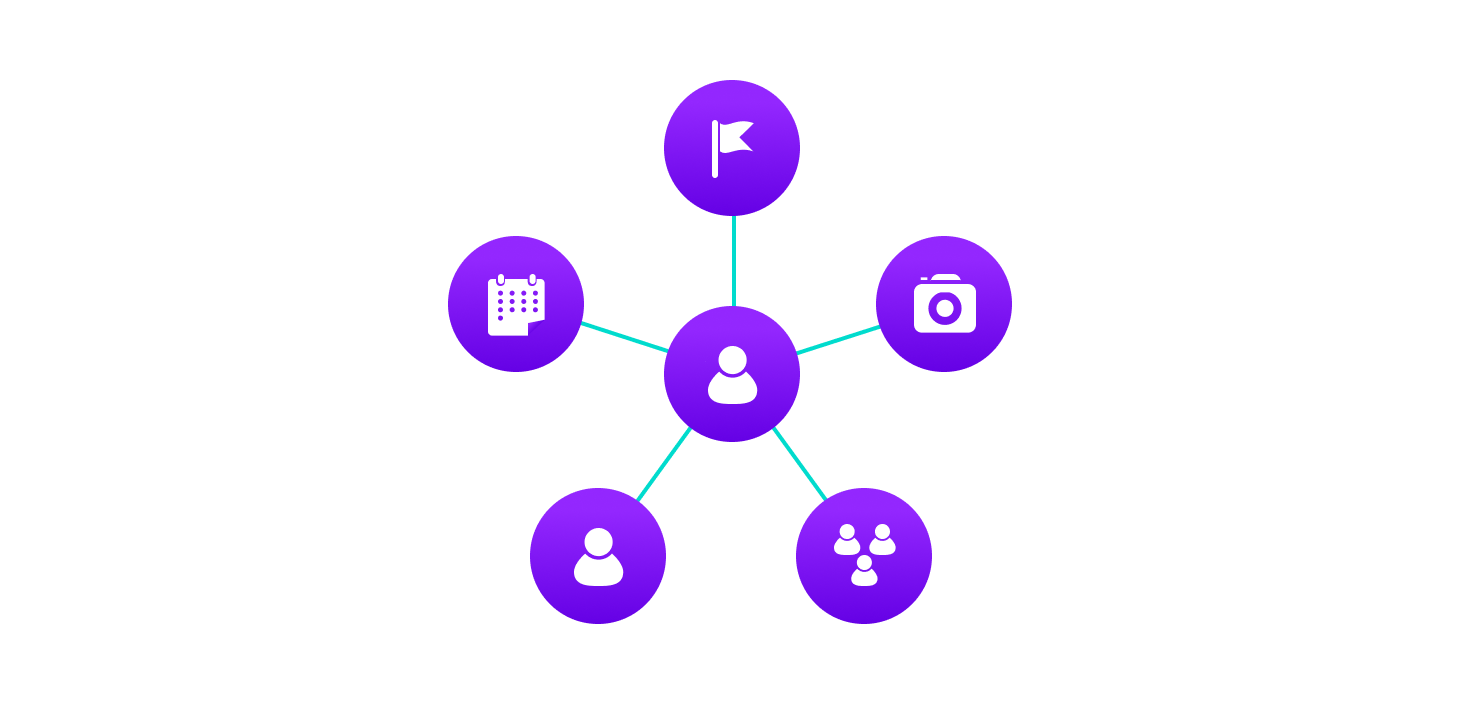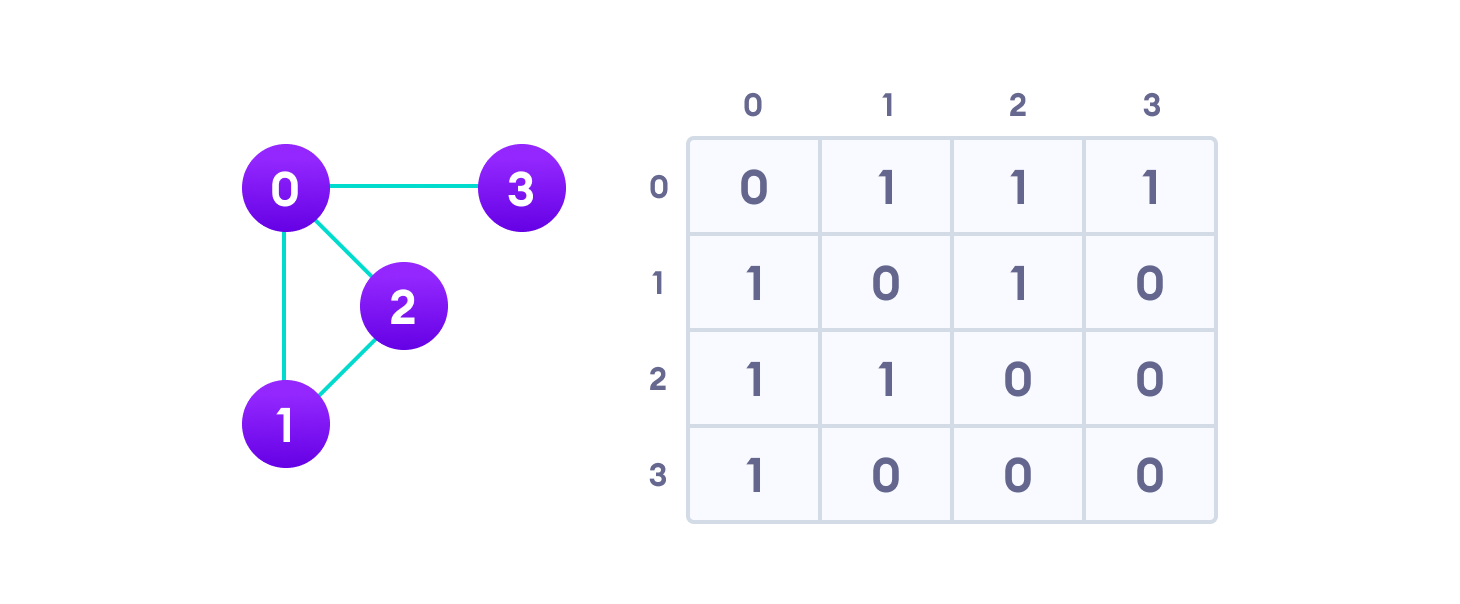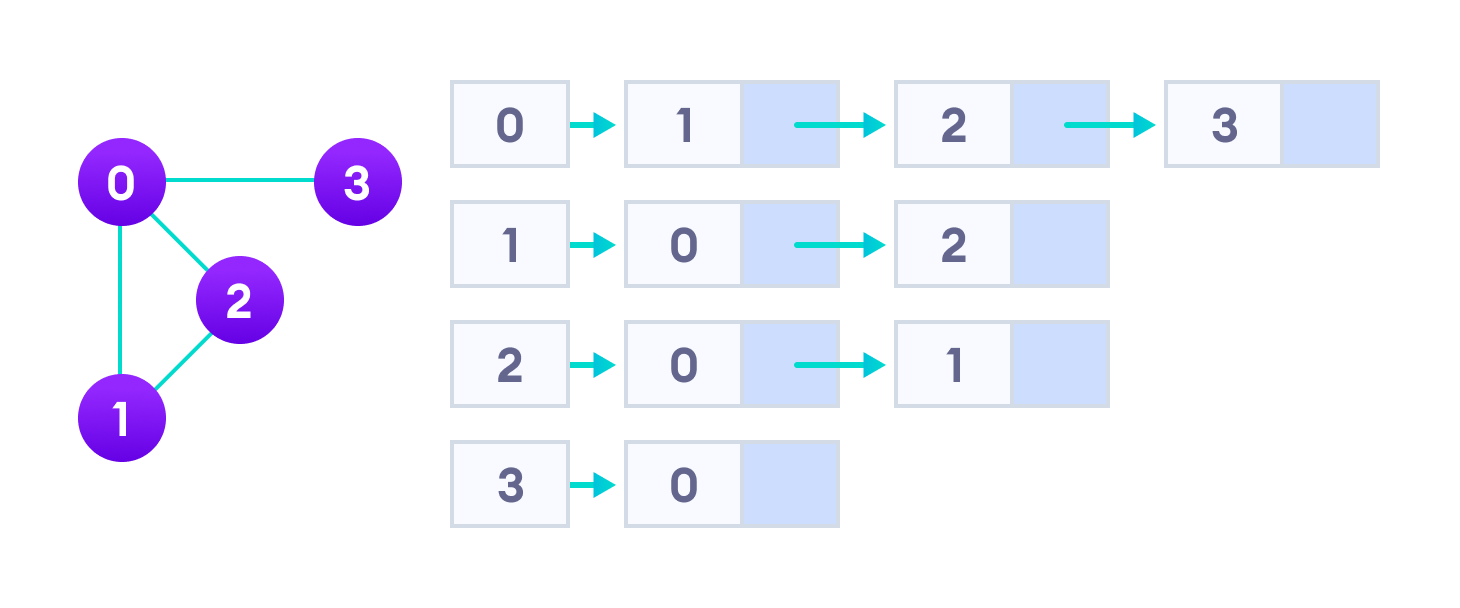Hits: 1

# Graph Data Structure

#### In this tutorial, you will learn what a Graph Data Structure is. Also, you will find representations of a graph.

A graph data structure is a collection of nodes that have data and are connected to other nodes.

Let’s try to understand this through an example. On facebook, everything is a node. That includes User, Photo, Album, Event, Group, Page, Comment, Story, Video, Link, Note…anything that has data is a node.

Every relationship is an edge from one node to another. Whether you post a photo, join a group, like a page, etc., a new edge is created for that relationship.Example of graph data structure

All of facebook is then a collection of these nodes and edges. This is because facebook uses a graph data structure to store its data.

More precisely, a graph is a data structure (V, E) that consists of

• A collection of vertices V
• A collection of edges E, represented as ordered pairs of vertices (u,v)Vertices and edges

In the graph,

```V = {0, 1, 2, 3}
E = {(0,1), (0,2), (0,3), (1,2)}
G = {V, E}```

## Graph Terminology

• Adjacency: A vertex is said to be adjacent to another vertex if there is an edge connecting them. Vertices 2 and 3 are not adjacent because there is no edge between them.
• Path: A sequence of edges that allows you to go from vertex A to vertex B is called a path. 0-1, 1-2 and 0-2 are paths from vertex 0 to vertex 2.
• Directed Graph: A graph in which an edge (u,v) doesn’t necessarily mean that there is an edge (v, u) as well. The edges in such a graph are represented by arrows to show the direction of the edge.

## Graph Representation

Graphs are commonly represented in two ways:

### 1. Adjacency Matrix

An adjacency matrix is a 2D array of V x V vertices. Each row and column represent a vertex.

If the value of any element `a[i][j]` is 1, it represents that there is an edge connecting vertex i and vertex j.

The adjacency matrix for the graph we created above isGraph adjacency matrix

Since it is an undirected graph, for edge (0,2), we also need to mark edge (2,0); making the adjacency matrix symmetric about the diagonal.

Edge lookup(checking if an edge exists between vertex A and vertex B) is extremely fast in adjacency matrix representation but we have to reserve space for every possible link between all vertices(V x V), so it requires more space.

### 2. Adjacency List

An adjacency list represents a graph as an array of linked lists.

The index of the array represents a vertex and each element in its linked list represents the other vertices that form an edge with the vertex.

The adjacency list for the graph we made in the first example is as follows:Adjacency list representation

An adjacency list is efficient in terms of storage because we only need to store the values for the edges. For a graph with millions of vertices, this can mean a lot of saved space.

## Graph Operations

The most common graph operations are:

• Check if the element is present in the graph
• Graph Traversal
• Add elements(vertex, edges) to graph
• Finding the path from one vertex to another

# Special 95% discount

## 2000+ Applied Machine Learning & Data Science Recipes

### Portfolio Projects for Aspiring Data Scientists: Tabular Text & Image Data Analytics as well as Time Series Forecasting in Python & R## Two Machine Learning Fields

There are two sides to machine learning:

• Practical Machine Learning:This is about querying databases, cleaning data, writing scripts to transform data and gluing algorithm and libraries together and writing custom code to squeeze reliable answers from data to satisfy difficult and ill defined questions. It’s the mess of reality.
• Theoretical Machine Learning: This is about math and abstraction and idealized scenarios and limits and beauty and informing what is possible. It is a whole lot neater and cleaner and removed from the mess of reality.

Data Science Resources: Data Science Recipes and Applied Machine Learning Recipes

Introduction to Applied Machine Learning & Data Science for Beginners, Business Analysts, Students, Researchers and Freelancers with Python & R Codes @ Western Australian Center for Applied Machine Learning & Data Science (WACAMLDS) !!!

Latest end-to-end Learn by Coding Recipes in Project-Based Learning:

Applied Statistics with R for Beginners and Business Professionals

Data Science and Machine Learning Projects in Python: Tabular Data Analytics

Data Science and Machine Learning Projects in R: Tabular Data Analytics

Python Machine Learning & Data Science Recipes: Learn by Coding

R Machine Learning & Data Science Recipes: Learn by Coding

Comparing Different Machine Learning Algorithms in Python for Classification (FREE)

`Disclaimer: The information and code presented within this recipe/tutorial is only for educational and coaching purposes for beginners and developers. Anyone can practice and apply the recipe/tutorial presented here, but the reader is taking full responsibility for his/her actions. The author (content curator) of this recipe (code / program) has made every effort to ensure the accuracy of the information was correct at time of publication. The author (content curator) does not assume and hereby disclaims any liability to any party for any loss, damage, or disruption caused by errors or omissions, whether such errors or omissions result from accident, negligence, or any other cause. The information presented here could also be found in public knowledge domains.  `